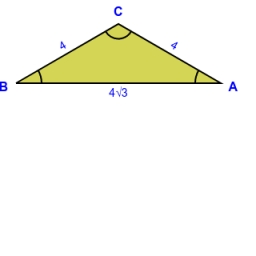# Two chords

From the point on the circle with a diameter of 8 cm, two identical chords are led, which form an angle of 60°. Calculate the length of these chords.

Correct result:

t =  6.928 cm

#### Solution:

$D=8 \ \text{cm} \ \\ A=60 \ ^\circ \ \\ B=A/2=60/2=30 \ ^\circ \ \\ r=D/2=8/2=4 \ \text{cm} \ \\ \ \\ r^2=r^2 + t^2 - 2 \cdot \ r \cdot \ t \cdot \ \cos B \ \\ t^2=2 \cdot \ r \cdot \ t \cdot \ \cos B \ \\ t=2 \cdot \ r \cdot \ \cos B ^\circ =2 \cdot \ r \cdot \ \cos 30^\circ \ =2 \cdot \ 4 \cdot \ \cos 30^\circ \ =2 \cdot \ 4 \cdot \ 0.866025=6.928 \ \text{cm}$Our examples were largely sent or created by pupils and students themselves. Therefore, we would be pleased if you could send us any errors you found, spelling mistakes, or rephasing the example. Thank you!

Leave us a comment of this math problem and its solution (i.e. if it is still somewhat unclear...):Be the first to comment!Tips to related online calculators
Looking for help with calculating roots of a quadratic equation?
Cosine rule uses trigonometric SAS triangle calculator.

#### You need to know the following knowledge to solve this word math problem:

We encourage you to watch this tutorial video on this math problem:

## Next similar math problems:

• WasteHow many percents are waste from a circular plate with a radius of 1 m from which we cut a square with the highest area?
• Ratio of sidesCalculate the area of a circle that has the same circumference as the circumference of the rectangle inscribed with a circle with a radius of r 9 cm so that its sides are in ratio 2 to 7.
• Quarter circleWhat is the radius of a circle inscribed in the quarter circle with a radius of 100 cm?
• DecagonCalculate the area and circumference of the regular decagon when its radius of a circle circumscribing is R = 1m
• NonagonCalculate the area and perimeter of a regular nonagon if its radius of inscribed circle is r = 10cm
• 30-gonAt a regular 30-gon the radius of the inscribed circle is 15cm. Find the "a" side size, circle radius "R", circumference, and content area.
• BillboardRectangular billboard is 2.5 m long with a diagonal 2.8 m long. Calculate the perimeter and the content area of the billboard.
• Circular poolThe base of the pool is a circle with a radius r = 10 m, excluding a circular segment that determines the chord length 10 meters. The pool depth is h = 2m. How many hectoliters of water can fit into the pool?
• Circular ringSquare with area 16 centimeters square are inscribed circle k1 and described circle k2. Calculate the area of circular ring, which circles k1, k2 form.
• Company logoThe company logo consists of a blue circle with a radius of 4 cm, which is an inscribed white square. What is the area of the blue part of the logo?
• Annular areaThe square with side a = 1 is inscribed and circumscribed by circles. Find the annular area.
• FlakesA circle was described on the square, and a semicircle above each side of the square was described. This created 4 "flakes". Which is bigger: the content of the central square or the content of four chips?
• Circular segmentCalculate the area S of the circular segment and the length of the circular arc l. The height of the circular segment is 2 cm and the angle α = 60°. Help formula: S = 1/2 r2. (Β-sinβ)Show that the quadrilateral with vertices P1(0,1), P2(4,2) P3(3,6) P4(-5,4) has two right triangles.Calculate the area of a rectangle with a diagonal of u = 12.5cm and a width of b = 3.5cm. Use the Pythagorean theorem.Calculate the perimeter and the area of square with a diagonal length 30 cm.The trapezium is formed by cutting the top of the right-angled isosceles triangle. The base of the trapezium is 10 cm and the top is 5 cm. Find the area of trapezium.